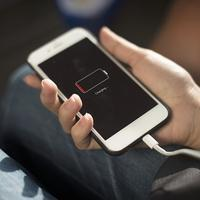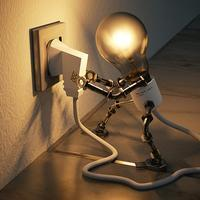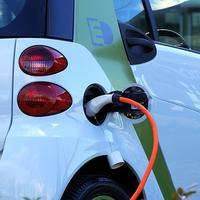# Electricity and magnetism

## What to expect

In this section you will learn about the big ideas of charge, a property that interacts with electric fields, electricity, the movement of charges in response to a force within a conductor, and magnetism, the forces exerted on magnetic domains or moving charges.

After studying this topic, you should be able to:

Studying at Higher Level? You'll also get to grips with EM induction (Faraday's lawLenz's lawAC generators and Transformers), rectification and capacitors.## Key questions

#### What exactly is an electric field?

An electric field is a region of space where a charge experiences a force. Find out more.

#### What is Coulomb's law?

Coulomb's law states that the force between two point charges is proportional to the product of their charge and inversely proportional to their separation squared. Find out more.

#### What is resistance and its formula?

Resistance is defined as the ratio of potential difference through a conductor to the current flowing through it. Find out more.

#### What are Kirchhoff's laws in electricity?

Kirchhoff's 1st law states that the sum of the currents into a junction is equal to the sum of the currents out. Kirchhoff's 2nd law states that, around any closed loop, the sum of the EMFs is equal to the sum of the potential differences. Find out more.

#### What is the test for a magnet?

To test for a magnet, you need to see if it can be repelled by an existing magnet. It is not enough to be attracted to an existing magnet, as all magentic materials are attracted to permanent magnets. Find out more.

#### How does an electromagnet work?

Moving charges (e.g. in any electric circuit!) produce a magnetic field. Find out more.

#### What are the principles of electromagnetic induction?

Faraday's law states that the magnitude of the induced EMF is proportional to the rate of change of magnetic flux. Find out more.

Lenz's law states that the direction of the induced current is such that it will oppose the change producing it. Find out more.

#### What is meant by rectification?

The simplest form of rectification is the insertion of a diode in the series circuit. All current in the forward direction is transmitted; no current flows in reverse bias. Full wave rectification uses two diode pathways (in opposite directions) to enable the current to flow at all times. Each diode path runs through the same resistor (or desired electrical component). Find out more.

#### What is the difference between a capacitor and capacitance?

Capacitors are electrical components used in circuits requiring timing or smoothing. They store charge, which is then released at a rate that can be controlled. The capacitance of a capacitor is defined as the ratio of the charge stored to the potential difference across the capacitor. Find out more.

•#### Electric fields

To understand electrical currents, we must first understand what an electric current consists of and what makes it 'go'.

•#### AHL Electric fields

In an electrical circuit, electrons move due to potential difference. Electric fields caused by charged plates, point charges or charged spheres cause lone charges to accelerate. This changes their energy.

•#### Electric circuits

Electric circuits need not be a source of worry. There are a lot of key words, but these can be defined and learned. There are a lot of possible arrangements, but these can be simplified. There appear to be several equations, but they all emerge from a few

•#### Magnetic fields

There are many questions you can expect to answer after studying Magnetism!

•#### AHL EM induction

Electromagnetic induction is the process by which kinetic energy is converted into electrical energy in the presence of a magnetic field.